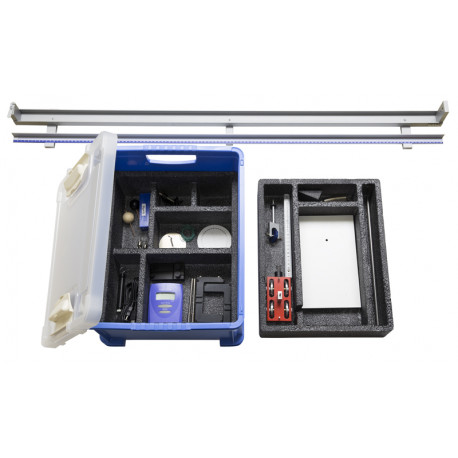# A3 DynamicsView larger
OPTIKA reserves the right to make corrections, modifications, enhancement, improvements and other changes to its products in any time without notice.
OPTIKA si riserva il diritto di apportare correzioni, modifiche, miglioramenti e altre modifiche ai suoi prodotti in qualsiasi momento senza preavviso.

A3

New

Dynamics

26 feasible experiments

Topics
• Motion
• Motion is relative
• Reference systems
• Trajectory
• Displacement
• Time table
• Tools for the experimental study of motion
• Manual time counting
• Automatic time counting
• Average speed
• How to measure the average speed
• Instantaneous velocity
• How to measure instantaneous velocity
• Average acceleration
• How to measure average acceleration
• Instantaneous acceleration
• Various types of motion
• Uniform rectilinear motion
• Uniformly accelerated rectilinear motion
• Uniformly accelerated motion: how to study it
• Causes of the motion
• When no forces are applied to a body
• When a constant force is applied to a body
• Taking stock of the work
• Mass
• The fundamental law of dynamics
• Energy conservation
• Free fall of a grave
• Periodic motions
• Simple pendulum
• Energy of an oscillating pendulum
• Gravity acceleration
• Springs properties
• Elastic pendulum